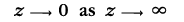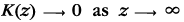# Errata for Microeconometrics Using Stata, Revised Edition

The errata for Microeconometrics Using Stata, Revised Edition are provided below. Click here for an explanation of how to read an erratum. Click here to learn how to determine the printing number of a book.

 (1) Chapter 2, p. 64, third line after (2.1)(1) Chapter 2, p. 67, second line after first displayed equation
 ... As the bandwidth parameter h increases, more... ... As the bandwidth parameter h increases, less...
 (1) Chapter 4, p. 120, end of first sentence of the second paragraph in section 4.2.1
 ... to produce a sequence of J integers between 0 and m. ... to produce a sequence of J integers between 0 and (m-1).
 (1,2) Chapter 4, p. 120, third sentence of the third paragraph in section 4.2.1
 The default is to have this set by Stata, based on the computer clock. The default is to have this set by Stata. Each time Stata is launched, the seed is set to 123456789. The state of the seed is updated each time a new random number is drawn.
 (1) Chapter 4, p. 137, second sentence of the third paragraph
 Then $$Y_{1}|Y_{2}\sim{N}\left\{ 0,(1-\rho^{2})\right\}$$ and $$Y_{2}|Y_{1}\sim{N}\left\{ 0,(1-\rho^{2})\right\}$$. Then $$Y_1 | Y_2 \sim N \left\{\rho Y_2 , ( 1 - \rho^2 ) \right\}$$ and $$Y_2 | Y_1 \sim N \left\{\rho Y_1 , ( 1 - \rho^2 ) \right\}$$.
 (1,2) Chapter 7, p. 213, in the sentence below (7.2), the definitions of A and B should be switched
 $$\mathbf{A}=\sum_{i}q(1-q)\mathbf{x}_{i}\mathbf{x}_{i}^{\prime}$$ $$\mathbf{A} =\sum_{i}f_{u_{q}}(0|\mathbf{x}_{i})\mathbf{x}_{i} \mathbf{x}_{i}^{\prime}$$ $$\mathbf{B}=\sum_{i}f_{u_{q}}(0|\mathbf{x}_{i})\mathbf{x}_{i} \mathbf{x}_{i}^{\prime}$$ $$\mathbf{B} =\sum_{i}q(1-q)\mathbf{x}_{i}\mathbf{x}_{i}^{\prime}$$